Ex 5.4 (Optional)

Chapter 5 Class 10 Arithmetic Progressions
Serial order wise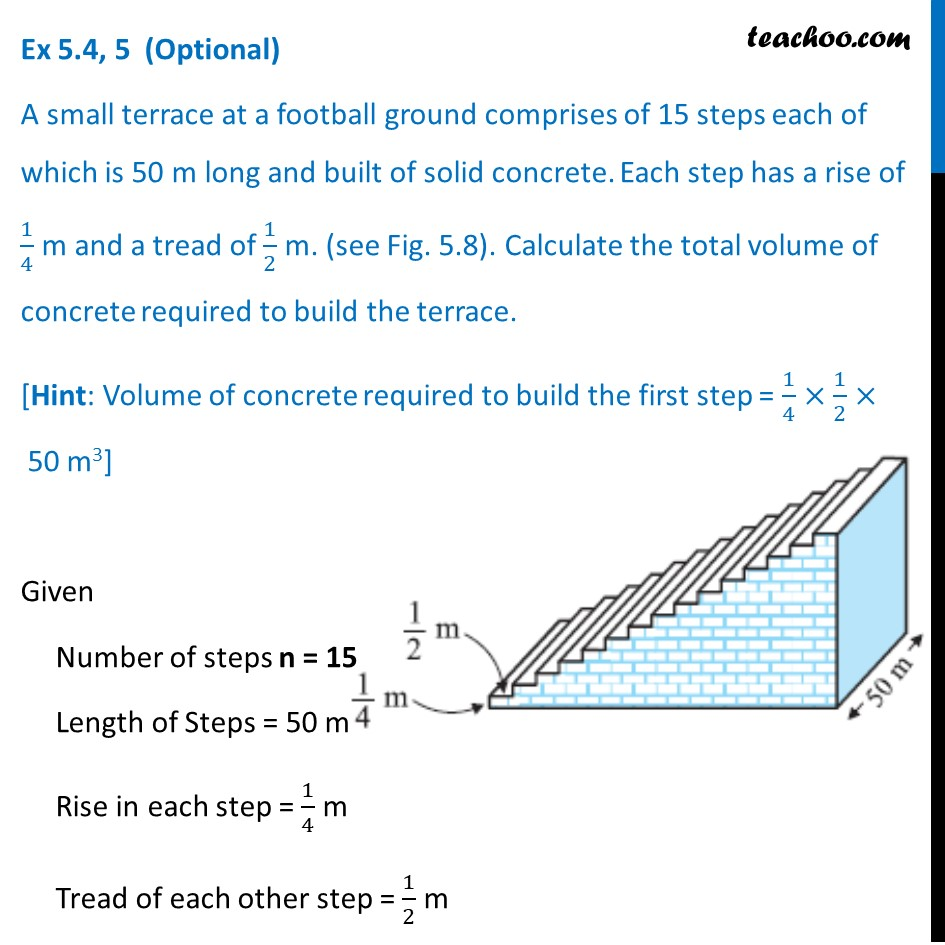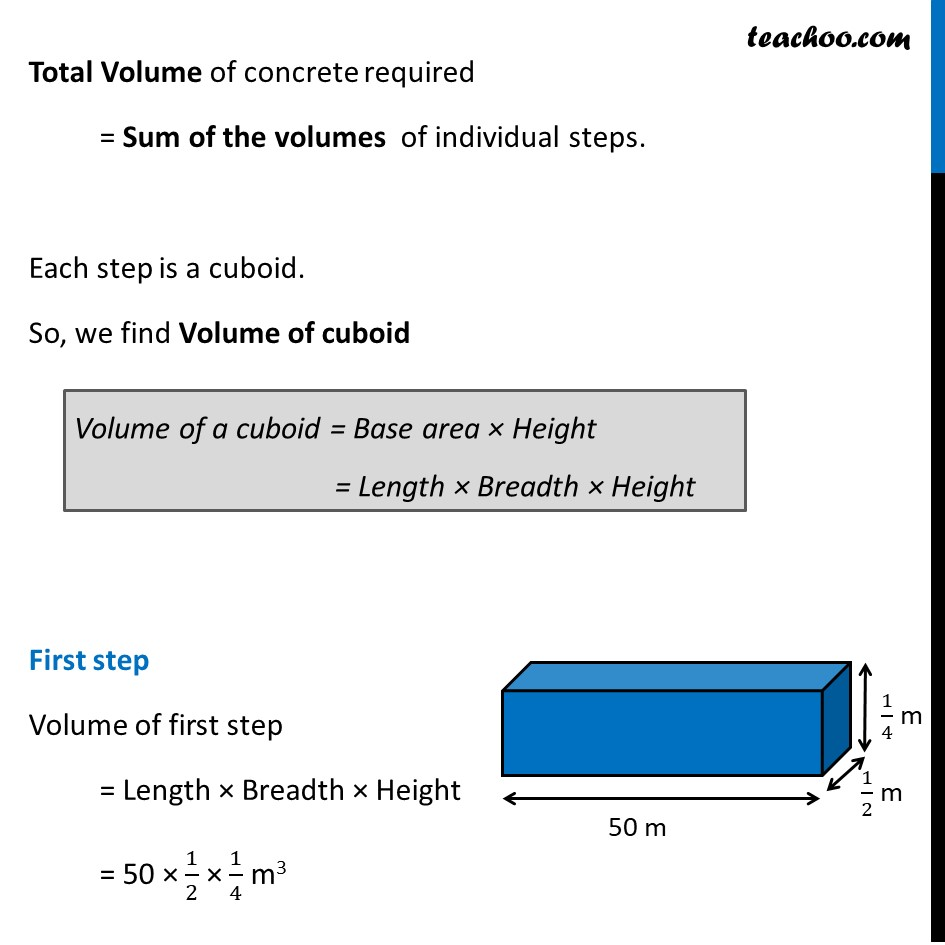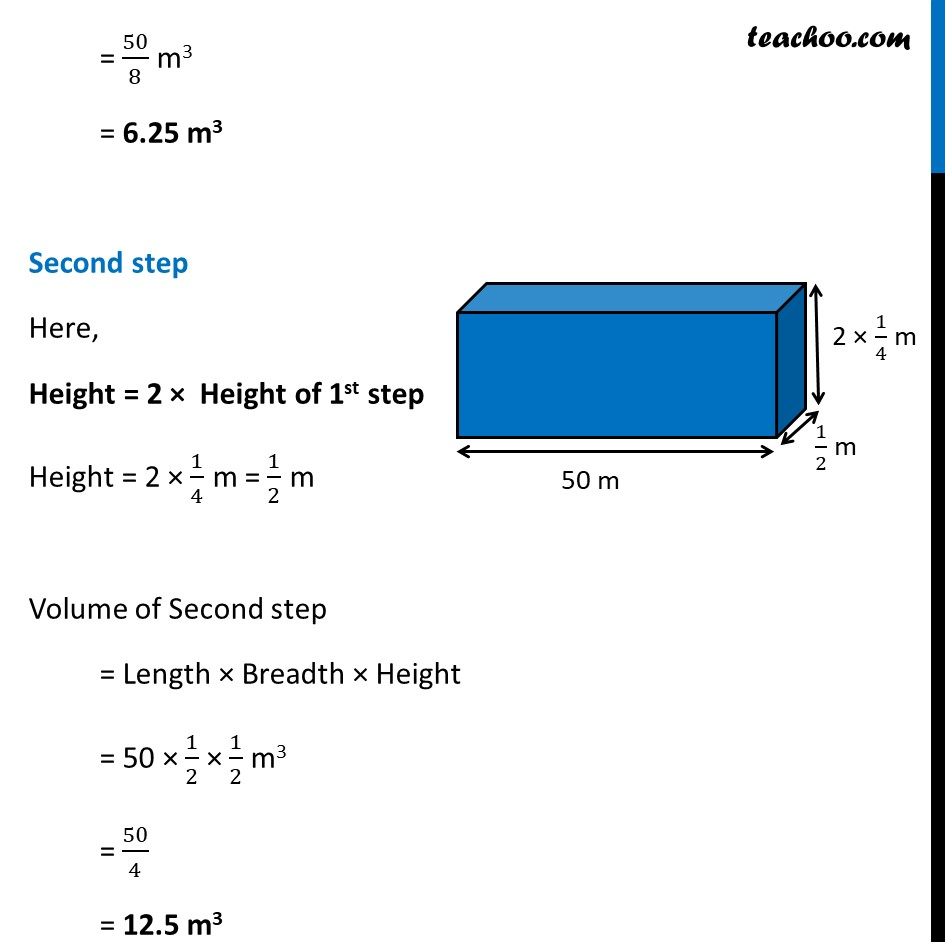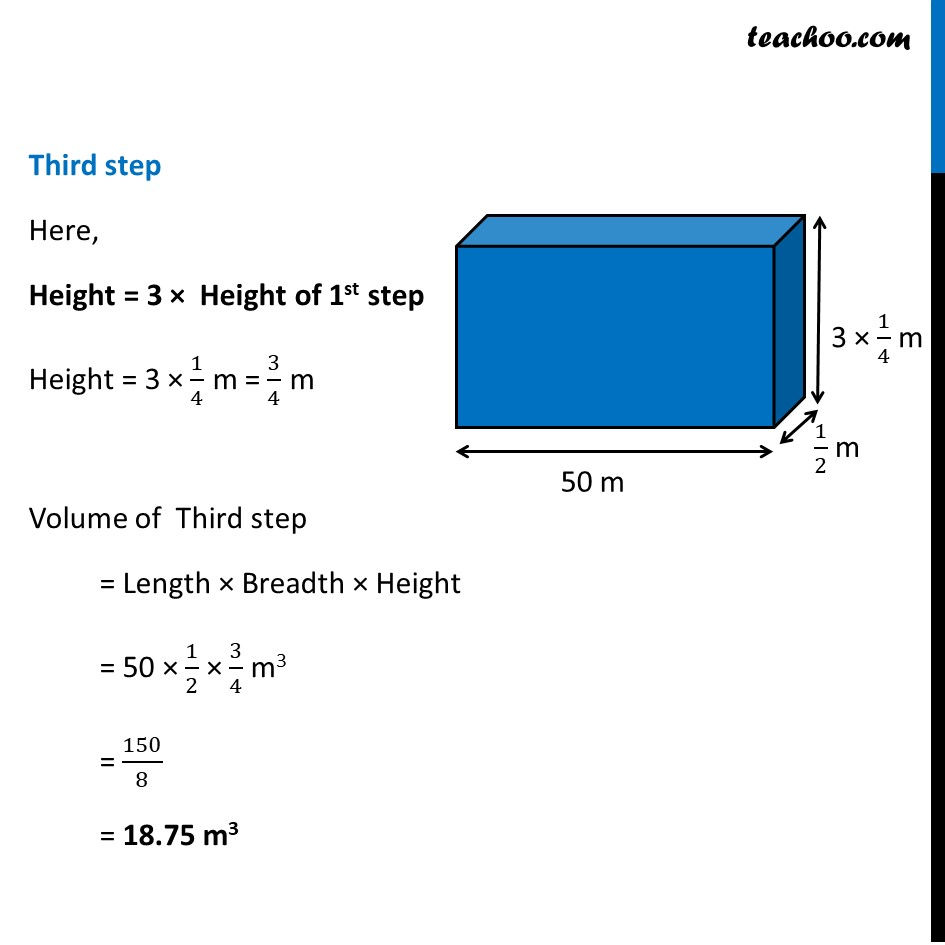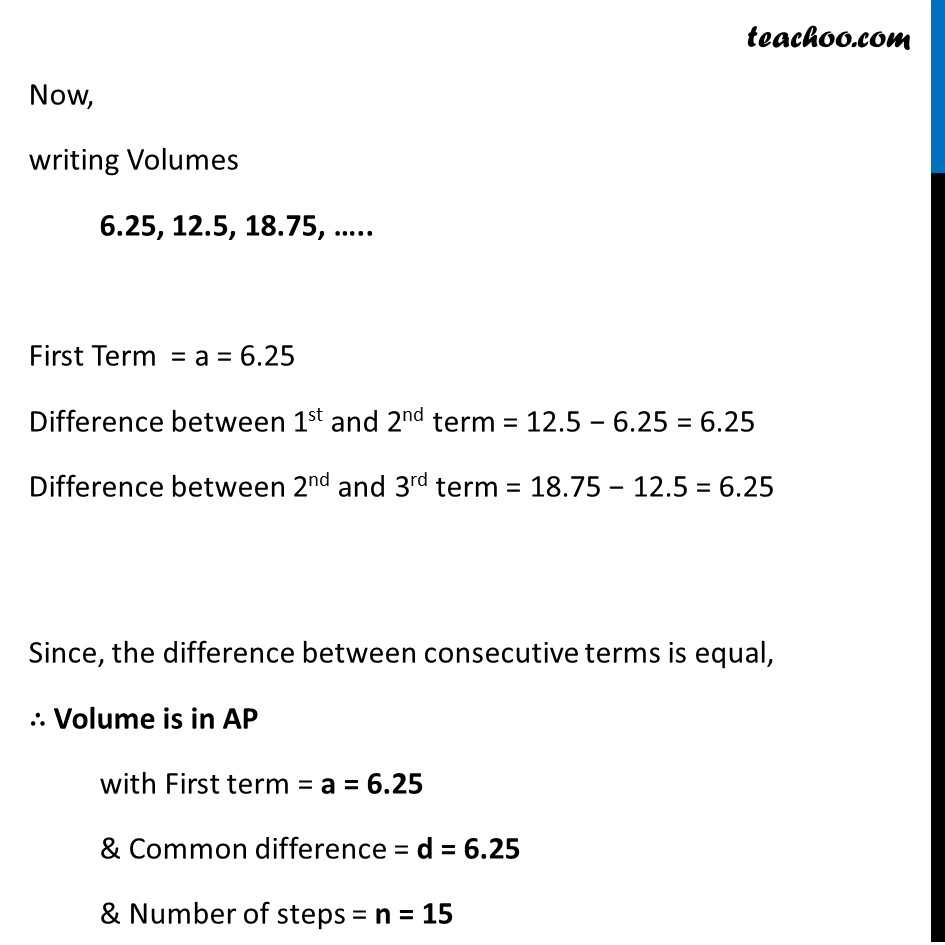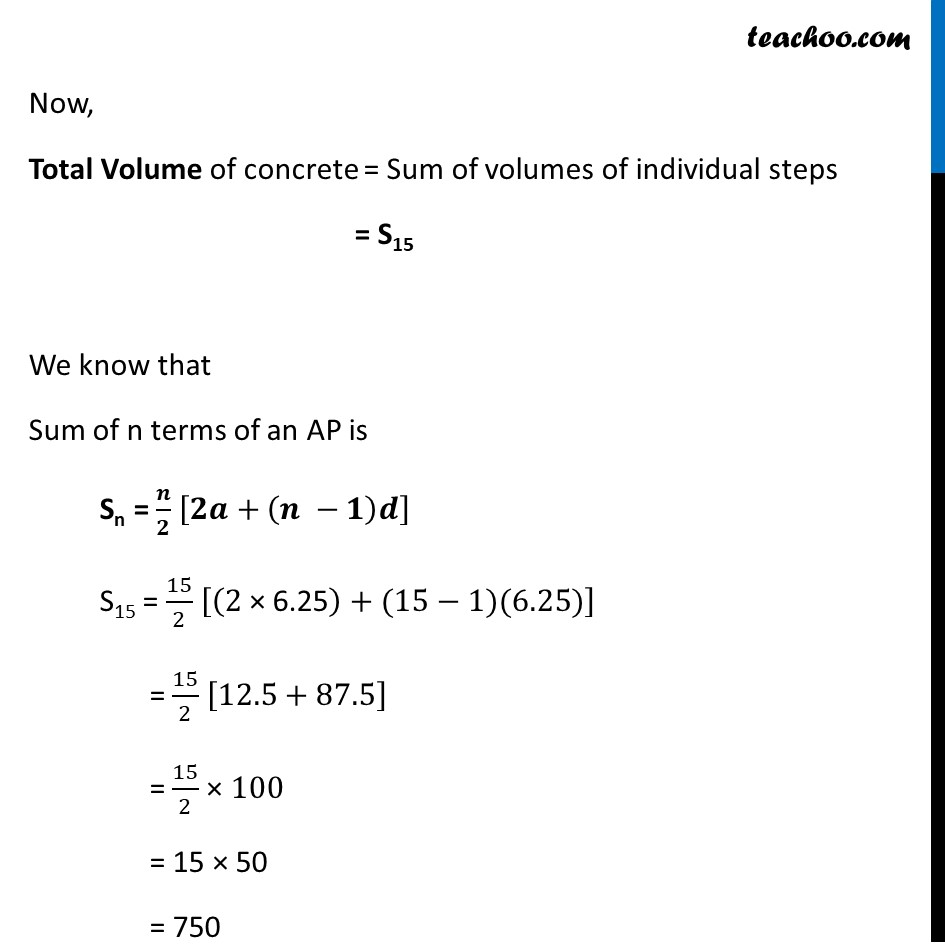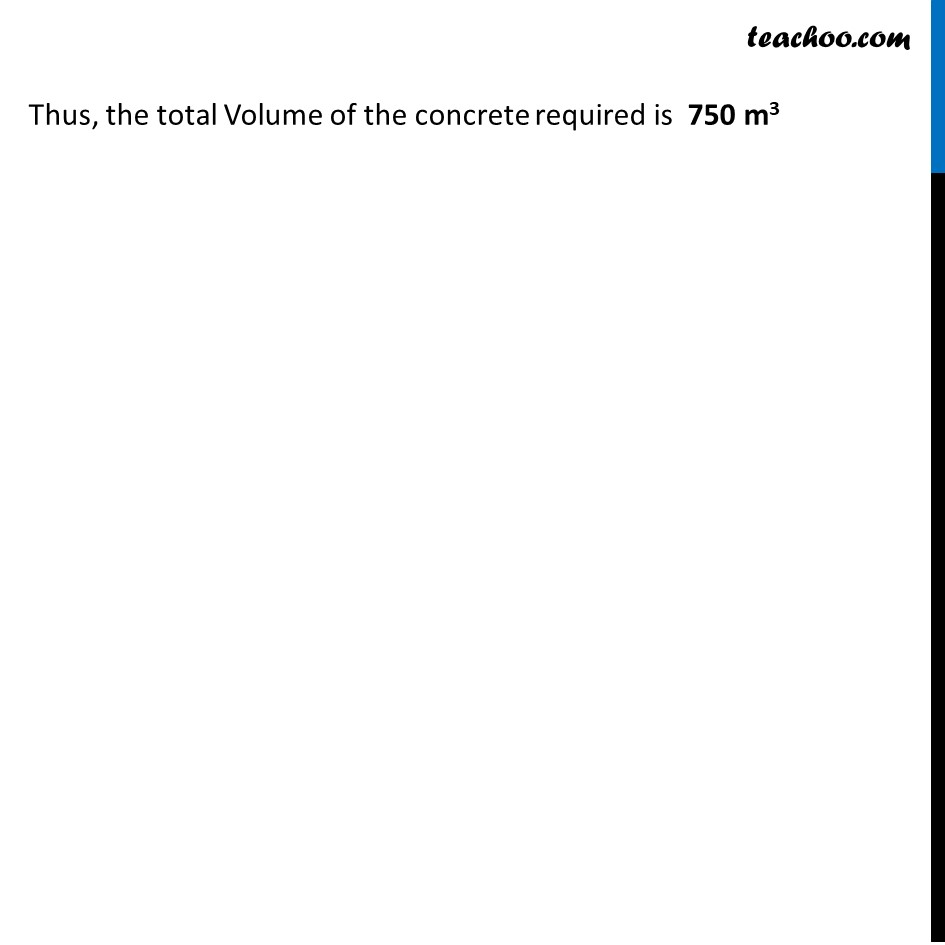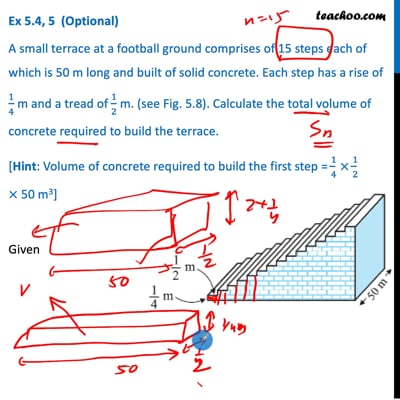This video is only available for Teachoo black users

Maths Crash Course - Live lectures + all videos + Real time Doubt solving!

### Transcript

Ex 5.4, 5 (Optional) A small terrace at a football ground comprises of 15 steps each of which is 50 m long and built of solid concrete. Each step has a rise of 1/4 m and a tread of 1/2 m. (see Fig. 5.8). Calculate the total volume of concrete required to build the terrace. [Hint: Volume of concrete required to build the first step = 1/4 × 1/2 × 50 m3] Given Number of steps n = 15 Length of Steps = 50 m Rise in each step = 1/4 m Tread of each other step = 1/2 m Total Volume of concrete required = Sum of the volumes of individual steps. Each step is a cuboid. So, we find Volume of cuboid First step Volume of first step = Length × Breadth × Height = 50 × 1/2 × 1/4 m3 Volume of a cuboid = Base area × Height = Length × Breadth × Height = 50/8 m3 = 6.25 m3 Second step Here, Height = 2 × Height of 1st step Height = 2 × 1/4 m = 1/2 m Volume of Second step = Length × Breadth × Height = 50 × 1/2 × 1/2 m3 = 50/4 = 12.5 m3 Third step Here, Height = 3 × Height of 1st step Height = 3 × 1/4 m = 3/4 m Volume of Third step = Length × Breadth × Height = 50 × 1/2 × 3/4 m3 = 150/8 = 18.75 m3 Now, writing Volumes 6.25, 12.5, 18.75, ….. First Term = a = 6.25 Difference between 1st and 2nd term = 12.5 − 6.25 = 6.25 Difference between 2nd and 3rd term = 18.75 − 12.5 = 6.25 Since, the difference between consecutive terms is equal, ∴ Volume is in AP with First term = a = 6.25 & Common difference = d = 6.25 & Number of steps = n = 15 Now, Total Volume of concrete = Sum of volumes of individual steps = S15 We know that Sum of n terms of an AP is Sn = 𝒏/𝟐 [𝟐𝒂+(𝒏 −𝟏)𝒅] S15 = 15/2 [(2" × 6.25" )+(15−1)(6.25)] = 15/2 [12.5+87.5] = 15/2 × 100 = 15 × 50 = 750 Thus, the total Volume of the concrete required is 750 m3